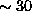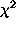Next: LTE Up: DESCRIPTION OF OBSERVATION-LEVEL PROGRAMS Previous: AO

## RATE_FIT

Purpose: To fit the observation data set light curves in-s intervals in seven broad pulse-height bands. The light curves are assumed to consist of a flat cosmic component, the calibrated particle background component, a linear scaling of the scattered solar X-ray component computed by the program AO, and a polynomial (up to fourth-order) describing the long-term enhancement background component. The constant cosmic background component is fit as part of the polynomial. The linear scale factor for the scattered solar X-rays (first-order polynomial coefficients) and the fourth-order polynomial coefficients of the long-term enhancement plus cosmic background components are fit by the program.

File Input:
1) Observation data set fits files - none
2) Other fixed input - valid_times_all.dat from the program VALID_TIMES, RATE1.DAT and RATE2.DAT from the program RATE, AO_SSX_SC.OUT from the program AO.

Interactive Input:
1) Band control - input 1-7 for pulse-height bands R1-R7. For this program, selecting 1 will serve for either band R1 or band R1L, as selected by the gain control of the program RATE (i.e., for the gain parameter 1 band R1 is produced, for the gain parameter 2 or 3, band R1L is produced).
2) Guess for the constant coefficient of the fourth-order polynomial - if the guess is non-zero, the program will query whether the coefficient should be fixed (enter 1) or not (enter 0). If the guess is zero, the program fixes the parameter at zero.
3-6) Guesses for the remaining coefficients of the polynomial - see 2) above.
7) Guess for the constant coefficient of the first-order polynomial for the scattered solar X-ray background - see 2) above.
8) Guess for the linear coefficient of the first-order polynomial for the scattered solar X-ray background - see 2) above.

Screen Output:
The program lists time offset (T0) and the values for the fitted parameters (I0 - I4 for the fourth-order polynomial and IS0 and IS1 for the linear scale factor) along with the formal uncertainties andstatistics. Note that a statistically-significantis not necessary. The goal is to get a reasonable fit to the general variation of the light curve, not to model the detailed variations (e.g., see the description for RATE). The values I0 - I4 will be used in the program LTE while the values IS0 and IS1 will be used in the program TILT. T0 will be used in both.

Ascii Output:
1) A QDP plot file is written with the name plot_n.qdp where n refers to the band which was fit. This plot should be examined to verify the quality of the fit and to identify possible short-term background enhancements that should be excluded. The specific times of background enhancements can be identified by using the RATE1.DAT and RATE2.DAT, which have the S/C time and count rates in the individual bands.Next: LTE Up: DESCRIPTION OF OBSERVATION-LEVEL PROGRAMS Previous: AO

Michael Arida
Tue Jul 30 15:18:52 EDT 1996